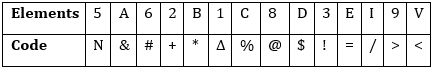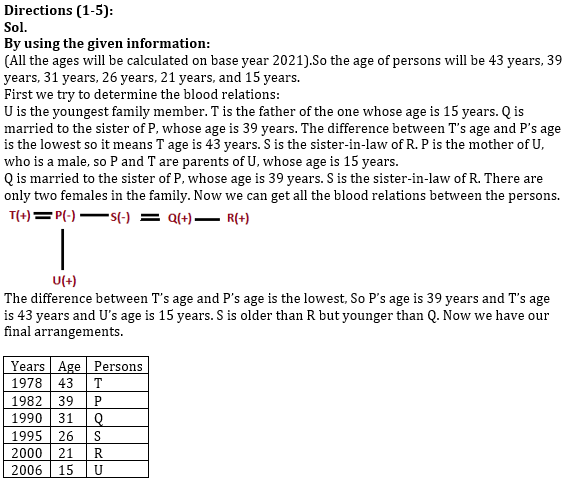Latest Banking jobs   »   Reasoning Ability Quiz For RRB PO...

# Reasoning Ability Quiz For RRB PO Mains 2021- 2nd September

Directions (1-5): Read the following information carefully and answer the following questions.
Six persons P, Q, R, S, T, and U are born in same month and date of six different year viz. 1978, 1982, 1990, 1995, 2000, and 2006 but not necessarily in the same order. (All the ages will be calculated on base year 2021). All persons are related to each other. T is the father of the one whose age is 15 years. The difference between T’s age and P’s age is the lowest. P is the mother of U, who is a male. Q is married to the sister of P, whose age is 39 years. S is the sister-in-law of R. S is older than R but younger than Q. There are only two females in the family. U is the youngest family member.

Q1.How is S related to the one whose age is 15 years?
(a) Paternal Uncle
(b) Maternal Uncle
(C) Maternal Aunt
(d) Paternal Aunt
(e) None of these

Q2. Who was born in 1990?
(a) S
(b) R
(C) Q
(d) T
(e) Either (b) or (c)

Q3. What is the sum of the ages of S and R?
(a) 52 years
(b) 57 years
(c) 47 years
(d) 60 years
(e) None of these

Q4. Which of the following information is correct?
(a) R was born in 1995
(b) R is the brother of Q
(C) Both (a) and (b)
(d) U is the son of P and T
(e) Both (b) and (d)

Q5. Four of the following five are alike in a certain way based on a group, which of the following does not belong to that group?
(a) T- 1978
(b) P -1982
(C) Q – 1990
(d) R- 1995
(e) U- 2006

Directions (6-10): In each of the questions given below, a group of digits is given followed by four combinations of letters/ symbols numbered. You have to find out which of the four combinations correctly represents the group of digits based on the letter/ symbol codes and the conditions given below. If none of the four combinations represents the group of digits correctly, give (e) i.e. ‘None of these’ as the answer.Condition for coding the group elements:
(i). If the first number is even and last letter is vowel, then the codes for the first and the last digits are to be interchanged.
(ii). If the first as well as the last letter is consonant, then both are to be coded by the code for the fourth letter.
(iii). If the first as well as the last number is odd, then both are to be coded by the code for the last number.
(iv). If the first number is odd and last letter is consonant, then both are to be coded by the code for the first number.

Q6. 5A8DCB9
(a) >&@\$*%>
(b) N&@\$%*>
(c) >&@\$%*N
(d) >&@\$%*>
(e) None of these

Q7. E31VI8D
(a) \$!∆</@\$
(b) =!∆/<@\$
(c) =!∆</@\$
(d) =!∆</@!
(e) None of these

Q8. C9AV32B
(a) %>&<!+<
(b) <>&<!+<
(c) <<&<!+<
(d) %>&<!+*
(e) None of these

Q9. 8A9D1IE
(a) =&\$>∆/@
(b) =&>\$∆=
(c) @&>\$∆=
(d) =&>\$∆/@
(e) None of these

Q10. 3BD8ACV
(a) !*\$@%&!
(b) !*\$@&%!
(c) !*\$*&%!
(d) !*#@&%!
(e) None of these

SolutionsS1. Ans. (c)

S2. Ans. (c)

S3. Ans. (c)

S4. Ans. (e)

S5. Ans. (d)

S6. Ans.(d)
Sol. Condition (iii) applies

S7. Ans.(c)
Sol. None of the conditions are applied.

S8. Ans.(b)
Sol. Condition (ii) applies

S9. Ans.(d)
Sol. Condition (i) Applies.

S10. Ans.(b)
Sol. Condition (iv) Applies.

Click Here to Register for Bank Exams 2021 Preparation Material#### Congratulations!Download Hindu Review of October 2021: Free PDF